# Microsoft Access Percentile Calculations with Total Access Statistics

Microsoft Access does not have a built-in Percentile function, so calculating percentiles has been challenging for MS Access users and developers. Fortunately, Total Access Statistics performs a wide range of percentile calculations on your Access tables and queries, with all results in Access tables that you can use in your queries, forms, and reports.

• Calculate different types of percentiles: median, quartiles, quintiles, octiles, deciles, percentiles, every X.5 percentile
• Results can be:
• Placed in an Access tables that you can use in your queries, forms, and reports
• Updated in a field in your table to assign the percentile value of the record
• Analyze the data in your Access tables and queries (including linked data)
• Support grouping on field values so you can perform multiple analyses in one pass

## Introduction to Percentiles

Percentiles are values based on rankings within a sorted list. The most common percentile is the median (50th percentile) which represents the middle value in a sorted list of values. For a normally distributed data set, this is identical to the mean (average) of all values, but if the data is skewed, the median may provide a more accurate description of the average (for example median home price is tracked rather than average home price which may be distorted by a few expensive home sales). The 0th percentile represents the smallest value and the 100th percentile represents the largest value.

## Percentile Features in Total Access Statistics

Total Access Statistics makes it easy to calculate the percentiles in Microsoft Access using data from any field from any table, linked table, or query:

• Specify the field(s) to calculate percentile values
• An unlimited number of records can be processed at one time
• Optionally specify Group Fields to calculate percentiles for each set of unique values in your Group Fields (similar to the way a GROUP BY field works in a SQL query)
• Optionally specify a weighting field to adjust the value of each record
• Optionally specify a value or range of values to ignore in the analysis

All the options can be selected interactively through the add-in Wizard interface. VBA developers can run the analysis through a programmatic VBA statistics function.

## Percentile Options in Total Access Statistics

There are several ways Total Access Statistics can calculate percentiles from your data. You can have the results in an output table with the percentile values as individual records or fields, or update a field in your table with the percentile value corresponding to the value in that record.

The primary Percentiles feature offers these output options: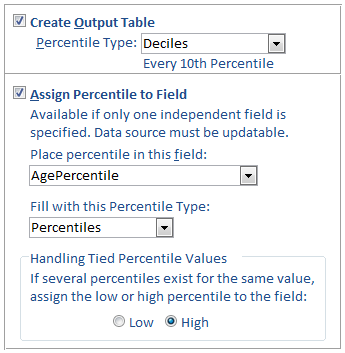### Option 1: Create an Output Table

A table is created containing the percentile values you specify. You can choose to create:

• Median for the middle value (50)
• Quartiles for 4 groups (25, 50, 75)
• Quintiles for 5 groups (20, 40, 60, and 80)
• Octiles for 8 groups (12.5, 25, 37.5, 50, 67.5, 75, and 87.5)
• Deciles for 10 groups (10, 20, 30, through 90)
• Percentiles for 100 groups (1, 2, through 99)
• X.5 Percentiles for 200 groups (0.5, 1, 1.5, through 99.5)

Every percentile option also generates the 0 and 100th percentiles (lowest and highest values). Here's an example of an output table: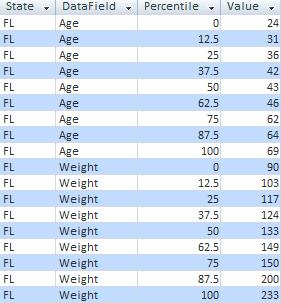Example of Octile calculations for Age and Weight fields Grouped by State Field (additional records for other states not shown)

### Option 2: Assign Percentile to a Field in Your Table

Instead of creating a table with the percentile results, you can save the percentile value directly in a field in your table. This lets you specify the percentile in the same record as your actual value.

The example on the right shows the field [AgePercentile] updated with the percentile value of the Age field.

There is an option to specify which percentile to assign to a record if the record’s value equals multiple percentiles. For instance, there may be several records tied for the highest value (100th percentile). That value could represent the 96th to 100th percentile. By choosing to assign the low value, the record would be assigned 96 (no other records would be assigned 97 to 100). By choosing the High option, they would all be assigned 100, and there would be no 96-99.## Percentile Options under Describe Scenarios

Percentiles are also available under the Describe (Field Descriptive) feature. Describe lets you specify values such as range, variance, skewness, mode, t-test vs. mean, etc. In addition to being able to calculate non-percentile results, the differences from the Percentile feature are:

• Describe shows the percentile values in separate fields vs. records
• The Percentile (100 values) and X.5 percentile (200 values) are not available. These options are: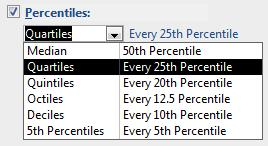### Percentile Calculations Under Describe

Here's an example of the output table from Describe calculating Quartiles on the Age field, grouped by State: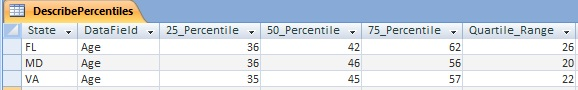## How Percentiles are Calculated

Percentiles are calculated by sorting the data from smallest to largest. The middle value is the median (50th percentile). Dividing into 4 groups gives quartiles (25th, 50th, and 75th percentiles), and 10 groups give deciles. This is the formula used to determine which record is selected for any percentile value: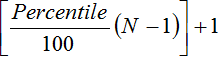where N is the number of items, and Percentile is a number between 0 and 100. For instance, for a sample size of 13, the quartile records for the 25th, 50th, and 75th percentiles are 4, 7, 11. By definition, the 0th and 100th percentiles are the lowest and highest values.

If the percentile cut-offs do not coincide with a particular data point, a linear interpolation of the two closest points is used. If a weighting field is assigned, values are considered continuous and no interpolation is performed.

## Interactive Wizard and VBA Programmatic Interfaces

Total Access Statistics lets you interactively generate your calculations through its wizard interface to easily select the data source, fields, and analysis without programming. After you specify your selections, they are automatically saved as a scenario in your database so you can run them again in the future when your data changes.

A VBA statistics function is also available so you can generate these results from code. You can easily run any saved scenario by calling a single procedure in the Total Access Statistics VBA library. The library includes a royalty-free runtime license so you can include it with your Access database and distribute it to non-Total Access Statistics owners.

Call the function from a button's OnClick event or other process, and the results are generated for you. You can even hide the built-in user interface so your users don't even know Total Access Statistics is running. They'll be amazed with your statistical analysis capabilities!

## Additional Details of Calculations Available in Total Access Statistics

Here are some additional resources and details about some of the data analysis you can perform on your Microsoft Access data with Total Access Statistics:

## Total Access Statistics

### Microsoft Access 2016 and 2013 Versions are shipping!

Version 14.1 for
Microsoft Access 2010

Version X.9 for
Microsoft Access 2007 and 2003

Version X.8 for
Microsoft Access 2002, 2000, and 97

### Reviews

"Total Access Statistics performs a wide range of data analysis right inside Access. The product includes a Statistics Wizard to perform parametric, group, non-parametric, record analysis and more. The results are placed in Access tables that you can integrate into your forms and reports."

Alison Balter, Author

More Awards Reviews and References in Scientific Papers

### Statistics InfoPDF Factsheet